# Bihar Board 12th Chemistry Model Question Paper 3 in English Medium

Bihar Board 12th Chemistry Model Papers

## Bihar Board 12th Chemistry Model Question Paper 3 in English Medium

Time : 3 Hours 15 Min
Full Marks: 70

Instructions for the candidates

1. Candidates are required to give answers in their own words as far as practicable.
2. Figures in the right hand margin indicates full marks.
3. While answering the questions, candidate should adhere to the words limit as far as practicable.
4. 15 Minutes of extra time has been allotted for the candidates to read the questions carefully.
5. This question paper is divided into two sections : Section -A and Section-B
6. In Section A, there are 35 objective type questions which are compulsory, each carrying 1 mark. Darken the circle with blue/black ball pen against the correct option on OMR Sheet provided to you. Do not use Whitener/Liquid/Blade/ Nail on OMR Sheet otherwise your result will be treated as invalid.
7. In section-B, there are 18 short answer type questions (each carrying 2 marks), out of which only 10 (ten) questions are to be answered Apart from this there are 06 Long Answer type questions (each carrying 5 marks), out of which 3 questions are to be answered.
8. Use of any electronic device is prohibited.

Objective Type Questions

In the following questions no. from 1 to 35, there is only one correct answer against each question. For each question, mark (darken) the correct answer on the OMR Sheet provided to you. (1 x 35 = 35)

Question 1.
Solid X is a very hard solid which is electrical insulator in solid as well as in molten state and has extremely high melting point. What type of solid is it ?
(a) Ionic solid
(b) Covalent solid
(c) Metallic solid
(d) Molecular solid
(b) Covalent solid

Question 2.
Examples of few solids are given below. Find out the example which is not correctly matched.
(a) Ionic solids – NaCl, ZnS
(b) Covalent solids – H2,I2
(c) Molecular solids – H2O(s)
(d) Metallic solids – Cu, Sn
(b) Covalent solids – H2,I2

Question 3.
Which of the following solids is the structure of CsCI crystal ?
(a) Body centred cubic
(b) Simple cubic
(c) Face centred cubic
(d) Edge centred cubic
(a) Body centred cubicQuestion 4.
Which of the following does not represent a type of crystal system ?
(a) Triclihic
(b) Monoclinic
(c) Rhombohedral
(d) Isotropical
(d) Isotropical

Question 5.
Which of the following primitive cells show the given parameters ?
a ≠ b ≠ c, α = β = γ = 90°
(a) Cubic
(b) Tetragonal
(c) Orthorhombic
(d) Hexagonal
(c) Orthorhombic

Question 6.
In amalgam of mercury with sodium, solvent is
(a) mercury
(b) sodium
(c) amalgam
(d) none of these
(b) sodium

Question 7.
What is the mole fraction of glucose in 10% w/W glucose . solution ?
(a) 01
(b) 0.02
(c) 03
(d) 0.04
(a) 01Question 8.
The cell reaction of the galvanic cell: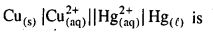(a) Hg + Cu2+→ Hg2+ + Cu
(b) Hg + Cu2+→ Cu+ + Hg+
(c) Cu + Hg → CuHg
(d) Cu + Hg2+ → Cu2+ + Hg
(d) Cu + Hg2+ → Cu2+ + Hg

Question 9.
Which of the following reaction is possible at anode ?
(a) 2Cr3+ +7H2O → Cr2O22-+14H+
(b) F2 → 2F
(c) (1/2)O2 +2H+→H2O
(d) None of these.
(a) 2Cr3+ +7H2O → Cr2O22-+14H+

Question 10.
Consider the reaction :$$-\frac{\mathrm{d}\left[\mathrm{N}_{2} \mathrm{O}_{4}\right]}{\mathrm{dt}}=\mathrm{k} \text { and } \frac{\mathrm{d}\left[\mathrm{NO}_{2}\right]}{\mathrm{dt}}=k^{\prime}$$
(a) 2 k’ = k
(b)k’ = 2k
(c)k’=k
(d) K = 1/4 k’
(b)k’ = 2kQuestion 11.
For the reaction 2NH3 → N2+3H2, if
$$-\frac{\mathrm{d}\left[\mathrm{NH}_{3}\right]}{\mathrm{dt}}=\mathrm{k}_{1}\left[\mathrm{NH}_{3}\right], \frac{\mathrm{d}\left[\mathrm{N}_{2}\right]}{\mathrm{dt}}$$ then the relation between k1, k2 and k3 is
(a) k1=k2 = k3
(b) k1=3k2-2k2
(c) 1.5= 3k2 = k3
(d) 2kx =k2= 3k3
(c) 1.5= 3k2 = k3

Question 12.
The term activation of adsorbent is used when
(a) adsorbing power is increased by increasing surface area by making the surface rough
(b) adsorbing power is increased by dipping the surface in acid to make it smooth
(c) adsorbing power is increased by dissolving it in water
(a) adsorbing power is increased by increasing surface area by making the surface rough

Question 13.
Which of the following is less than zero during adsorption ?
(a) ΔG
(b) ΔS
(c) ΔH
(d) All of these
(d) All of these

Question 14.
In which of the following the name of the ore is not matched with its formula ?
(a) Cassiterite – SnO2
(b) Limonite – Fe2O3.3H2O
(c) Siderite – FeCO3
(d) Anglesite – PbCO3
(d) Anglesite – PbCO3

Question 15.
Pyrolusite is ………………
(a) a sulphide ore of Mn
(b) an oxide ore of Mn
(c) a carbide ore of P
(d) a chloride ore of Zn
(b) an oxide ore of Mn

Question 16.
Nitrogen forms stable N2 molecule but phosphorus is converted to.P4 from P2 because
(a) pπ – pπ bonding is strong in phosphorus
(b) pπ – pπ bonding is weak in phosphorus
(c) triple bond is present in phosphorus
(d) single P – P bond is weaker than N – N bond
(b) pπ – pπ bonding is weak in phosphorusQuestion 17.
The decreasing order of boiling points of the following hydrides is
(a) H2O > SbH3 > AsH3 > PH3 > NH3
(b) H2O > NH3 > SbH3 > AsH3 > PH3
(c) H2O>SbH3>NH3>AsH3>PH3
(d) H2O > PH3 > AsH3 > SbH3 > NH3
(c) H2O>SbH3>NH3>AsH3>PH3

Question 18.
Which of the following pairs of ions have the same electronic configuration ?
(a) Cu2+,Cr2+
(b) Fe3+,Mn2+
(c) CO3++Ni3+
(d) SC3+ + cr 3+
(b) Fe3+,Mn2+

Question 19.
The melting points of Cu, Ag and Au follow the order
(a) Cu > Ag > Au
(b) Cu > Au > Ag
(c) Au > Ag > Cu
(d) Ag > Au > Cu
(b) Cu > Au > Ag

Question 20.
When one mole of each of the following complexes is treated with excess of AgN03, which will give maximum amount of AgCl ?
(a) [Co(NH3)6]Cl3
(b) [Co(NH3)5Cl]Cl2
(c) [Co(NH3)4Cl2]Cl
(d) [Co(NH3)3Cl3]
(a) [Co(NH3)6]Cl3

Question 21.
According to Werner’s theory of coordination compounds.
(a) primary valency is ionisable
(b) secondary valency is ionisable
(c) primary and secondary valencies are ionisable
(d) neither primary nor secondary valency is ionisable
(a) primary valency is ionisable

Question 22.
Haloalkanes contain halogen atom(s) attached to the sp3 hybridised carbon atom of an alkyl group. Identify haloalkane from the following compounds.
(i) 2-Bromopentane
(ii) Vinyl chloride
(iii) 2-Chloroacetophenone
(iv) Trichloromethane
(a) Only (ii)
(b) (ii) and (iv) only
(c) (i) and (iv) only
(d) (i), (ii) and (iii) only
(c) (i) and (iv) onlyQuestion 23.
The IUPAC name of tertiary butyl chloride is ……………..
(a) 2-chloro-2-methypropane
(b) 3-chlorobutane
(c) 4-chlorobutane
(d) 1, 2-chloro-3-methylporpane .
(a) 2-chloro-2-methypropane

Question 24.
A compound …………. has two tertiary alcoholic groups. The IUPAC name of this compound is
(a) 2, 3-dimethyl-1, 2-butanediol
(b) 3, 3-dimethyl-1, 2-butanediol
(c) 2, 3-dimethyl-2, 3-butanediol
(d) 2-methyI-2, 3-pentanediol
(c) 2, 3-dimethyl-2, 3-butanediol

Question 25.
Which of the following is phenol ?
(a) Cresol
(b) Catechol
(c) Benzenol
(d) All of these
(d) All of these

Question 26.
Ketones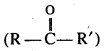can be obtained in one step by (where R and R’ are alkyl groups)
(a) hydrolysis of esters
(b) oxidation of primary alcohols
(c) oxidation of secondary alcohols
(d) reaction of alkyl halides with alcohols
(c) oxidation of secondary alcohols

Question 27.
Ozonojysis of an organic compound gives formaldehyde as one of the products. This confirms the presence of
(a) two ethylenic double bonds
(b) a vinyl group
(c) an isopropyl group
(d) an acetylenic triple bond
(b) a vinyl groupQuestion 28.
Which of the following is not a primary amine ?
(a) tert-Butylamine
(b) sec-Butylamine
(c) Isobutylamine
(d) Dimethylamine
(d) Dimethylamine

Question 29.
Which one of the following is aromatic amine ?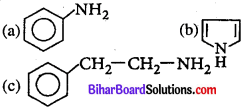(d) Both (a) and (b)
(d) Both (a) and (b)

Question 30.
Cellulose is a
(a) hexapolysaccharide
(b) pentapolysaccharide
(c) tripolysaccharide
(d) none of these
(d) none of these

Question 31.
Which of the following is a non-reducing sugar ?
(a) Glucose
(b) Sucrose
(c) Maltose
(d) Lactose
(b) Sucrose

Question 32.
Which of the following sets contain only addition homopolymers ?
(a) Polythene natual rubber, cellulose
(b) Nylon polyester, melamine resin
(c) Teflon, bakelite, orlon
(d) Neoprene, PVC, polythene
(d) Neoprene, PVC, polythene

Question 33.
Teflon and neoprene are the examples of
(a) copolymers
(b) monomers
(c) homopolymers
(d) condensation polymers
(c) homopolymers

Question 34.
Some drugs do not bind to the enzyme’s active site, instead bind to a different site of enzyme. This site is called
(a) allosteric site
(b) substrate site
(c) ionic site
(d) competitive site.
(a) allosteric siteQuestion 35.
Drugs that bind to the receptor site and inhibit its natural function are called
(a) agonistic drugs
(b) antagonistic drugs
(c) antimicrobial drugs
(d) allosteric drugs.
(b) antagonistic drugs

Non-Objective Type Questions

In this Section, there are 18 Short answer type questions (each carrying 2 marks), out of which answer any 10 questions.  2 x 10 = 20

Question 1.
What happens when
(a) ethanol is oxidised with acidified KMnO4 solution ?
(b) ethanol is treated with PCl5 ?
(a) Ethanoic acid will be formed(b) Chloroethane will be formed
C2H5OH + PCl5 → C2H5Cl + POCl3 + HCl

Question 2.
How will you convert
(a) aniline to 2, 4, 6 tribromoaniline ?
(b) acetamide to ethylamine ?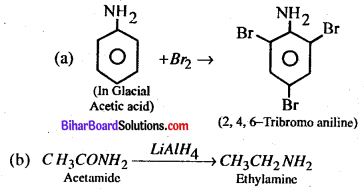Question 3.
(a) What is inert pair effect ?
(b) Why does BCI3 act as Lewis acid ?
Inert pair effect – The pairs of two electrons doesn’t participated in a chemical reaction of heavy outside & subnucleic non metal. This effect is called inert pair effect. BCl3 behaves as Lewis acid due to the electron deficient in BCl3

Question 4.
Explain the following :
(a) H2S is a gas while H2G is a liquid at room temperature.
(b) Carbon and silicon are tetravalent but Ge, Sn and Pb show divalency also.
(a) Since H – 0 bond is more polar than H – S because of greater electronegativity difference in the bond participating atoms. Due to this, H2O molecules are involved in the bending associates to be liquid at room temperature.

Where as, the intermolecular hydrogen bonding is negligible in the molecules of H2S and does not associates, and it maintains the gaseous state at room temperature.

(b) Due to small shape of atoms of carbon and silicon and due to the maximum value of electron negativity. The carbon and silicon are tetravalent but the Sn, Pb shows divalency.Question 5.
Write the IUPAC names of the following coordinate compounds :
(a) [Pt (NH3)2 Cl2] Cl2
(b) [Cr (NH3)4 Cl2]+
(a) [Pt (NH3)2Cl2[Cl2 → Diammine dicholorplatinum (iv) chloride.
(b) [Cr {NH3)4Cl2]+ → Tetra aminedichloro chromium (iii) ion.

Question 6.
(a) Why are Cd2+ salts white ?
(b) Why does H3PO3 act as reducing agent but H3PO4 does not ?
(a) Due to absence of unpaired electron CD2+ molecule is found CD2+ salt in white.
(b) The oxidation no. of P in H3PO3 in +3 so its oxidation no. can be increased, or we can say that H3PO3 acts as reducing agent while the oxidation no. of P is +5 in H3 PO4 . Therefore, we can say that H3PO3 acts as reducing agent but H3PO4 does not act as reducing agent.

Question 7.
Write IUPAC names of the following :
(a) [Cu (NH3)4]Cl2
(b) K4 [Fe (CN)6 ]
(a) Hexa-amino Copper (II) Chloride
(b) Potassium hexacyano Ferrate (II)

Question 8.
How will you convert the following ?
(a) Ethyl alcohol to Ethylene
(b) Acetylene to Benzene.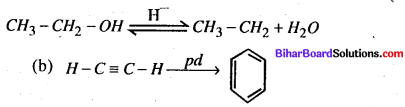Question 9.
How will you account for the following ? The electron affinity of fluorine is less than that of chlorine.
The electron affinity of fluorine is less than that of chlorine because atom of chlorine is much smaller, hence electron density is very high, so an external electron can be added easily.

Question 10.
Give reasons for the following :
(a) Formic acid is stronger that Acetic acid.
(b) Aniline is less basic than methylamine.
(a) Acidity of acid depends upon donation of proton
Since $$K a=\frac{\left[R C O O^{-}\right]\left[H_{3} O^{+}\right]}{[R C O O H]}$$
As we know, if the value of Ka is max, The Acidity will be maximum
HCOOH (Formic acid) ⇒ Ka= 1.77 x 10 5
CH3COOH+ (acidic acid) ⇒ Ka = 1.75 x 105
Hence formic acid is more acidic than acetic acid.(b) One pair of electron of nitrogen atom in aniline, which is overlaped with re-electron in ring. So, nitrogen atoms is positive charged or their is lack of electrons and it isn’t in the state than it can give paired electron to acid in comparison with methyl amine, due to this reason there is no overlapping so, aniline is a weak base than methyl amine.

Question 11.
Define the following : (a) Roasting (b) Smelting.
Roasting – Roasting is the process by heating an ore in oxygen below its melting point to avoid volatile impurities.
Example –
CuS + 3O2 → 2Cm2 O+2SO2
S + O→ SO2
Smelting – Smelting is process in which metal oxide is obtained by heating with some reducing agent such metals are Zn, Fe, Sn, Cu.

Question 12.
Give two methods for the preparation of colloids.
There are two methods of the preparation of colloids

• Condensation method
• Disperson method

Question 13.
State coiligative properties of dilute solution. Write down the different types of coiligative properties.
The property, which depends upon number of atom or molecule of the solute and hot on their nature is called coiligative properties. There are four type of coiligative properties.

• Relative lowering of vapour pressure.
• Elevation in boiling point.
• Deperession in freezing point.
• Osmosis or Osmotic pressure.

Question 14.
The rate constant of a first order reaction is 60 sec-1. How much time will it take to reduce 75% of its initial concentration ?
Let initial concentration = amz 1; According to Question 75% reduce
Let after t second, concentration remains $$\frac{a}{4}$$
As we know $$t=\frac{2.303}{t} \log \frac{a}{a-x}$$
$$t=\frac{2.303}{60} \log 4$$log 4 = 0.023 second
t = 0.023 secondsQuestion 15.
Prove that the half-life of a first order reaction is independent of the initial concentration.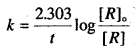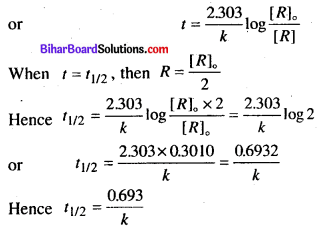From above, it is clear that the half-life of a first order reaction is independent of the initial concentration.

Question 16.
What do you mean by vapour pressure ? What happens to the vapour pressure. When (i) volatile solute is dissolved in solution, (ii) non-volatile solute is dissolved in solution ?
Vapour pressure : It is defined as the pressure exerted by a vapour in thermodynamic equilibrium with its condensed phased (solid or liquid) at a given temperature in a closed sytem.

• Volatile solute increases vapour pressure of solvent.
• Nonvolatile solute lowers vapour pressure of solvent.

Question 17.
Differentiate between thermoplastic and thermosetting polymers. Give one example of each.
Thermoplastic : Thermoplastic is a polymer that becomes pladable or moledable above a specific temperature and returns to a state upon cooling. Most thermoplastic have a high molecular weight.
Example : Polyethelene.

Thermosetting : A thermosetting is also known as thermoset is petrochemical material that irreversibly chures. The cure may be induced by heat through chemical reaction. A cured thermosetting resin is called a thermoset.
Example: Polyester.

Question 18.
How many moles of Cu will be deposited by passing 24125 coulombs of electric current from CuSQ4 solution ?
Cu++ + 2e → Cu
2 x 96500 = 1 mole
Change 2 x 96500 columb Cu heated on = 1 mole 24125 columb change Cu on
1 Mole x $$\frac{24125}{2 \times 96500}$$ = 0.125 mole

There are 06 long answer type questions (each carrying 05 marks), out of which answer any there questions.
(5×3 = 15)

Question 19.
(a) What is the difference between Ferromagnetism and paramagnetism ?
(b) For the cell shown below Zn (s) | ZnSO4 (aq) || CuSO4 (aq) | Cu (s) Calculate standard cell potential if standard state reduction electrode potentials for Cu2+ |Cu and Zn2+| Zn are + 0.34 V and – 0.76 V respectively.
(a) Paramagnetism :

• The substances which are weakly attracted by magnetic field are called paramagnetic substances.
• These substances have permanent magnetic dipoles due to the presence of atom or ions with
unpaired electrons.
• These substances lose their magnetism in the absence of magnetic field, e.g., TiO, CuO.

Ferromagnetism :

• The substances which are strongly attracted by magnetic field are called ferromagnetic substance.
• These substances show permanent magnetic even in the absence of magnetic field.
• e.g., Iron, Cobalt, Nickel & CrO2
Zn (s) \ZnSOI4(a2)\\CuSO4(aq)\Cu (s)
So, the cell reaction

At Anode : OxidationQuestion 20.
(a) What do you mean by the term elevation in boiling point’ ?
(b) State Raoult’s law. How is it applicable in determining the molecular weight of non-electrolyte solute ?
(a) Elevation in boiling – The boiling point of a liquid may be defined as the temperature at which its vapour pressure becomes equal to atmospheric pressure. When we add a solute in any pure solvent, then the boiling point of the solution gets increased. This increasement is known as the elevation in boiling point.

(b) Raoult’s law – According to this law, the vapour pressure of a solution containing non-volatile solute is directly proportional to the mole fraction of the solvent.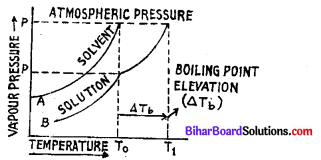Fig. Vapour pressure curve explaining elevation of boiling point. If a solution contains two components A (volatile solvent) and B (non-volatile solute) the vapour pressure of solution is given as
[Vapour Pressure of solution ] = [Vapour Pressure of solvent insolution (PA)] α [ Mole fraction of solvent (Xa)]

PA α XA where, K is a proportionality constant
PA = KXa
Roult’s law is applicable in determing the molecular weight of non-electrolyte solute because unsaturated solute in solvent is pure solute than its boiling point is elevated. Hence, by this law we determine the molecular weight of non-electrolyte solute.

Question 21.
(a) Give the principle for the manufacture of nitric acid from ammonia.
(b) Give the reaction of 50% dilute HNO3 with copper metal.
(a) Due to following 11 parts of NH3 and 89 part of mixture of air Pt gaize and heated at 100°, then we get (NO).
4NH3+ 5O2 → 4 NO + 6H2O
2NO + O2 → 2NO2
2N02 + H2O → HNO3 + HNO2
HNO2 → HNO3 +2NO+ H2O
Thus, from above equations it is clear that NO. gives NO2 due to its oxidation which mix in water and forms HNO3 .

(b) 3Cw + dil. SHNO3 → 3Cu(NO3)2 +4H2O + 2NO

Question 22.
What happens when
(a) Ammonia reacts with excess of chlorine ?
(b) H2S reacts with acidified K2Cr2O7 solution ?
(c) Copper sulphate reacts with KI Solution ?
(a) Ammonia reacts with excess of Chlorine
2NH3 + 6Cl2 → 2NCl3 + 6HCl

(b) When H2S reacts with acidified K2Cr2O7 solution then a milky white or pale yellow colour of solution appears, due to the oxidations of H2S to sulphur which are of colloidal nature.
H2S + K2Cr2O7 → K2SO4 + Cr2O3 + H2O

(c) When copper sulphate (CuSO4) reacts with KI solution, then a white precipitate of cuprous iodide is formed.
[CuSO4 +2KI → Cul2 + K2SO4] x 2
2CuI2 → CuI2 + I2
2CuSO4 + 4KI → 2K2SO4 + Cu2I2 + I2

Question 23.
(a) Describe the following chemicals with suitable examples :
(i) Antipyretics
(ii) Antiseptics.
(b) Name the vitamin whose deficiency is responsible for night blindness.
(c) What are the starting materials used for preparing bakelite and PVC ?
(a) (i) Antipyretics – Those chemicals which are used to lower down the body temperatures during high fever are known as antipyretics, e.g., Paracetamol, aspirin, etc.

Antiseptic – Those chemicals which are able to kill the micro-organisms, which are harmful to us are known as antiseptic. e.g., dettol or tincture of iodine can be applied an wounds, cuts, etc. They are also applied to reduce odours resulting from bacteria de-composition on the body.

(b) Vitamin A or A, is the vitamin whose deficiency is responsible for night blindness.

(c) Bakelite – Phenol and formaldehyde PVC – Methyl chlorideQuestion 24.
(a) Define the following with one example in each case : (i) Analgesics (ii) Antibiotics.
(b) How many molecules of ATP are produced per molecule of glucose in glycolysis ?
(c) What are synthetic rubbers ? Give one example.